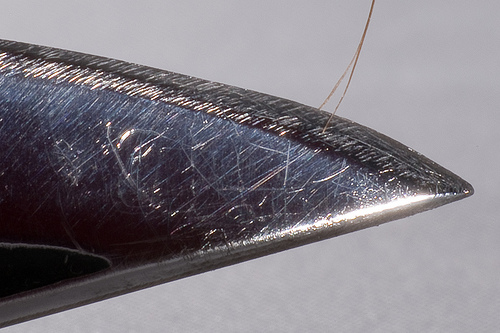I need to display a hexadecimal code 000102 on a lcd screen but it shows 012 Limited Time Offer
i use lcd.print

lcd.print(myHexVariable, HEX); will display whatever myHexVariable is in HEX, but if it isn't in HEX to begin with, it will be converted (aka if you have an integer, this would be stored in DEC and would then be converted to HEX which will not be the same thing).

If you make this myHexVariable, having 0x before your value will make it a hexadecimal.

myHexVariable = 0x000102.

However, even if you print it using the above described method, the '000' at the beginning will be cutoff due to being excess.

``````sprintf ( buf, "%06x", someLongValue);
``````

BTW, are we doing better or worse than the French speakers?

cypherrage:
lcd.print(myHexVariable, HEX); will display whatever myHexVariable is in HEX, but if it isn't in HEX to begin with, it will be converted (aka if you have an integer, this would be stored in DEC and would then be converted to HEX which will not be the same thing).

What? Hex and Decimal are just ways to visually represent the data using ASCII characters, it's all stored the same in variables:

``````int x = 123; // is the same as
int y = 0x7B; // which is also the same as
int z = 0b011110111; // this
``````

Arrch:

cypherrage:
lcd.print(myHexVariable, HEX); will display whatever myHexVariable is in HEX, but if it isn't in HEX to begin with, it will be converted (aka if you have an integer, this would be stored in DEC and would then be converted to HEX which will not be the same thing).

What? Hex and Decimal are just ways to visually represent the data using ASCII characters, it's all stored the same in variables:

``````int x = 123; // is the same as
``````

int y = 0x7B; // which is also the same as
int z = 0b011110111; // this

Apologies, I don't think anything I said was wrong, maybe it was just not said the right way.

``````int x = 123 is in DEC
int y = 0x7B is in HEX
int z = 0b011110111 is in BIN

lcd.print(x, HEX) gives 0x7B.
lcd.print(x, BIN) gives 0b011110111
``````

I unfortunately probably made it sound like all integers are "automatically decimal". If you do not specify a prefix (0x or B), it will be in decimal format (yes I realize it is stored as a character and not some arbitrary decimal variable) when doing math and printing. Thank you for clarifying.

If you do not specify a prefix (0x or B), it will be in decimal format

"If you do not specify a prefix (0x or B or 0b or 0), it will be in decimal format"

cypherrage:
I unfortunately probably made it sound like all integers are "automatically decimal". If you do not specify a prefix (0x or B), it will be in decimal format (yes I realize it is stored as a character and not some arbitrary decimal variable) when doing math and printing. Thank you for clarifying.

The problem is with the word stored.

``````int x = 123; // is the same as
int y = 0x7B; // which is also the same as
int z = 0b011110111; // this
``````

Are all stored the same way. The way they are printed, however, depends on the second argument (if supplied) and the variable type.

AWOL:

If you do not specify a prefix (0x or B), it will be in decimal format

"If you do not specify a prefix (0x or B or 0b or 0), it will be in decimal format"

Actually specifying a prefix of B it will still be in decimal format to the compiler - the preprocessor replaces Bxxxxx with a decimal valuekhallal:
I need to display a hexadecimal code 000102 on a lcd screen but it shows 012 Limited Time Offer
i use lcd.print

That could be either a hardware or software problem, but a software problems seems more likely.